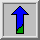# Roberts Cross Edge DetectorCommon Names: Roberts Cross

## Brief Description

TheRoberts Cross operator performs a simple, quick to compute, 2-D spatial gradient measurement on an image. It thus highlights regions of high spatial frequency which often correspond to edges. In its most common usage, the input to the operator is a grayscale image, as is the output. Pixel values at each point in the output represent the estimated absolute magnitude of the spatial gradient of the input image at that point.

## How It Works

In theory, the operator consists of a pair of 2×2 convolution kernels as shown in Figure 1. One kernel is simply the other rotated by 90°. This is very similar to the Sobel operator.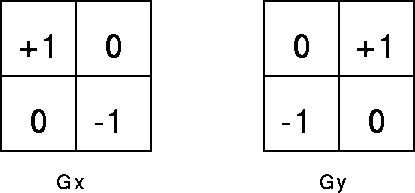Figure 1 Roberts Cross convolution kernels

These kernels are designed to respond maximally to edges running at 45° to the pixel grid, one kernel for each of the two perpendicular orientations. The kernels can be applied separately to the input image, to produce separate measurements of the gradient component in each orientation (call these Gx and Gy). These can then be combined together to find the absolute magnitude of the gradient at each point and the orientation of that gradient. The gradient magnitude is given by: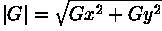although typically, an approximate magnitude is computed using: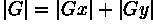which is much faster to compute.

The angle of orientation of the edge giving rise to the spatial gradient (relative to the pixel grid orientation) is given by:In this case, orientation 0 is taken to mean that the direction of maximum contrast from black to white runs from left to right on the image, and other angles are measured clockwise from this.

Often, the absolute magnitude is the only output the user sees --- the two components of the gradient are conveniently computed and added in a single pass over the input image using the pseudo-convolution operator shown in Figure 2.Figure 2 Pseudo-convolution kernels used to quickly compute approximate gradient magnitude

Using this kernel the approximate magnitude is given by: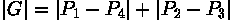## Guidelines for Use

The main reason for using the Roberts Cross operator is that it is very quick to compute. Only four input pixels need to be examined to determine the value of each output pixel, and only subtractions and additions are used in the calculation. In addition there are no parameters to set. Its main disadvantages are that since it uses such a small kernel, it is very sensitive to noise. It also produces very weak responses to genuine edges unless they are very sharp. The Sobel operator performs much better in this respect.

We useto illustrate the effect of the operator.

The imageis the corresponding output from the Roberts Cross operator. The gradient magnitudes output by the operator have been multiplied by a factor of 5 to make the image clearer. Note the spurious bright dots on the image which demonstrate that the operator is susceptible to noise. Also note that only the strongest edges have been detected with any reliability.

The imageis the result of thresholding the Roberts Cross output at a pixel value of 80.

We can also apply the Roberts Cross operator to detect depth discontinuity edges inrange images. In the range imagethe distance from the sensor to the object is encoded in the intensity value of the image. Applying the Roberts Cross yieldsThe operator produced a line with high intensity values along the boundary of the object. On the other hand, intensity changes originating from depth discontinuities within the object are not high enough to output a visible line. However, if we threshold the image at a value of 20, alldepth discontinuities in the object produce an edge in the image, as can be seen inThe operator's sensitivity to noise can be demonstrated if we add noise to the above range image. The image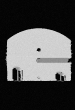is the result of adding Gaussian noise with a standard deviation of 8,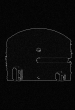is the corresponding output of the Roberts Cross operator. The difference to the previous image becomes visible if we again threshold the image at a value of 20, as can be seen in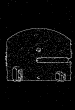Now, we not only detect edges corresponding to real depth discontinuities, but also some noise points. We can show that the Roberts Cross operator is more sensitive to noise than, for example, the Sobel operator if we apply the Sobel operator to the same noisy image. In that case, we can find a threshold which removes most of the noise pixels while keeping all edges of the object. Applying a Sobel edge detector to the above noisy image and thresholding the output at a value of 150 yields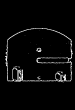The previous examples contained sharp intensity or depth changes, which enabled us (in the noise-free case) to detect the edges very well. The imageis a range image where the depth values change much more slowly. Hence, the edges in the resulting Roberts Cross image,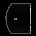are rather faint. Since the intensity of many edge pixels in this image is very low, it is not possible to entirely separate the edges from the noise. This can be seen in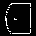which is the result of thresholding the image at a value of 30.

The effects of theshape of the edge detection kernel on the edge image can be illustrated usingApplying the Roberts Cross operator yieldsDue to the different width and orientation of the lines in the image, the response in the edge image varies significantly. Since the intensity steps between foreground and background are constant in all patterns of the original image, this shows that the Roberts Cross operator responds differently to different frequencies and orientations.

If the pixel value type being used only supports a small range of integers (e.g. 8-bit integer images), then it is possible for the gradient magnitude calculations to overflow the maximum allowed pixel value. In this case it is common to simply set those pixel values to the maximum allowed value. In order to avoid this happening, image types that support a greater range of pixel values, e.g. floating point images, can be used.

There is a slight ambiguity in the output of the Roberts operator as to which pixel in the output corresponds to which pixel in the input, since technically the operator measures the gradient intensity at the point where four pixels meet. This means that the gradient image will be shifted by half a pixel in both x and y grid directions.

## Interactive Experimentation

You can interactively experiment with this operator by clicking here.

## Exercises

1. Why does the Roberts Cross' small kernel size make it very sensitive to noise in the image?

2. Apply the Roberts Cross operator to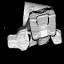Can you obtain an edge image that contains only lines corresponding to the contours of the object? Compare with the results obtained with the Sobel and Canny operators.

3. Compare the result of applying the Roberts Cross operator towith the one of using the Sobel operator.

4. Compare the performance of the Roberts Cross with the Sobel operator in terms of noise rejection, edge detection and speed.

5. Under what situations might you choose to use the Roberts Cross rather than the Sobel? And under what conditions would you avoid it?

## References

R. Boyle and R. Thomas Computer Vision: A First Course, Blackwell Scientific Publications, 1988, pp 50 - 51.

E. Davies Machine Vision: Theory, Algorithms and Practicalities, Academic Press, 1990, Chap. 5.

L. Roberts Machine Perception of 3-D Solids, Optical and Electro-optical Information Processing, MIT Press 1965.

D. Vernon Machine Vision, Prentice-Hall, 1991, Chap. 5.

## Local Information